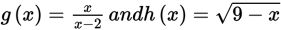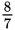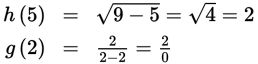# SAT Math Multiple Choice Question 917: Answer and Explanation

### Test Information

Question: 9171. Given the functions defined above, what is the value of (g ° h)(5)?

• A. 0
• B.• C. 2
• D. Undefined

Explanation:

D

Difficulty: Medium

Category: Passport to Advanced Math / Functions

Strategic Advice: The notation (g ° h)(x) indicates a composition of two functions, which can also be written as g(h(x)) and read as "g of h of x." It means that the output when x is substituted in h(x) becomes the input for g(x). Just remember that when working with compositions, you always start with the function that is closest to x.

Getting to the Answer: Substitute 5 for x in h(x), simplify, and then substitute the result into g(x):Be careful-a number divided by 0 is not 0! In fact, division by 0 is not defined, so (g ° h)(5) is undefined, which is (D).